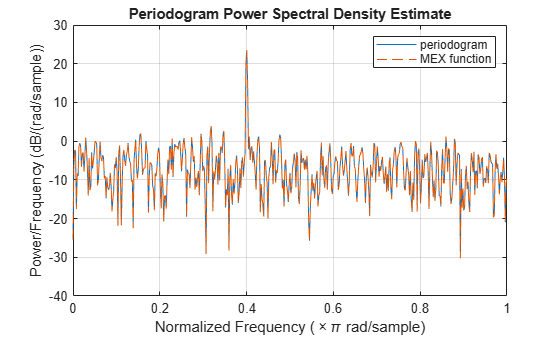# Compute Modified Periodogram Using Generated C Code

Create a function `periodogram_data.m` that returns the modified periodogram power spectral density (PSD) estimate of an input signal using a window. The function specifies a number of discrete Fourier transform points equal to the length of the input signal.

`type periodogram_data`
```function [pxx,f] = periodogram_data(inputData,window) %#codegen nfft = length(inputData); [pxx,f] = periodogram(inputData,window,nfft); end ```

Use `codegen` (MATLAB Coder) to generate a MEX function.

• The `%#codegen` directive in the function indicates that the MATLAB® code is intended for code generation.

• The `-args` option specifies example arguments that define the size, class, and complexity of the inputs to the MEX-file. For this example, specify `inputData` as a 1024-by-1 double precision random vector and `window` as a Hamming window of length 1024. In subsequent calls to the MEX function, use 1024-sample input signals and windows.

• If you want the MEX function to have a different name, use the `-o` option.

• If you want to view a code generation report, add the `-report` option at the end of the `codegen` command.

`codegen periodogram_data -args {randn(1024,1),hamming(1024)}`
```Code generation successful. ```

Compute the PSD estimate of a 1024-sample noisy sinusoid using the periodogram function and the MEX function you generated. Specify a sinusoid normalized frequency of $2\pi /5$ rad/sample and a Hann window. Plot the two estimates to verify they coincide.

```N = 1024; x = 2*cos(2*pi/5*(0:N-1)') + randn(N,1); periodogram(x,hann(N)) [pxMex,fMex] = periodogram_data(x,hann(N)); hold on plot(fMex/pi,pow2db(pxMex),':','Color',[0 0.4 0]) hold off grid on legend('periodogram','MEX function')```